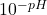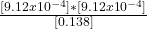## A monoprotic weak acid when dissolved in water is 0.66% dissociated and produces a solution with a pH of 3.04. Calculate the Ka of the acid.

Question

A monoprotic weak acid when dissolved in water is 0.66% dissociated and produces a solution with a pH of 3.04. Calculate the Ka of the acid. g

in progress 0
6 months 2021-07-26T12:26:08+00:00 1 Answers 24 views 0

## Answers ( )

Ka = 6.02×10⁻⁶

Explanation:

The equilibrium that takes place is:

• HA ⇄ H⁺ + A⁻
• Ka = [H⁺][A⁻]/[HA]

We calculate [H⁺] from the pH:

• pH = -log[H⁺]
• [H⁺] =• [H⁺] = 9.12×10⁻⁴ M

Keep in mind that [H⁺]=[A⁻].

As for [HA], we know the acid is 0.66% dissociated, in other words:

• [HA] * 0.66/100 = [H⁺]

We calculate [HA]:

• [HA] = 0.138 M

Finally we calculate the Ka:

• Ka == 6.02×10⁻⁶